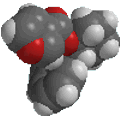Useful ConceptsNomenclature Summary / Group Priority

This section contains the basic IUPAC rules for nomenclature and a table of functional groups in priority order, with examples.

For a complete introduction to organic nomenclature : Basic IUPAC Organic Nomenclature

Naming branched chain alkanes (the IUPAC way....)

• Find the parent hydrocarbon. This is based on the longest continuous carbon chain. If there are chains of equivalent length, then select the one with the most branch points as the parent.
• Number the atoms in the parent chain, beginning at the end nearer the first branch point. If there is branching an equal distance from both ends, then consider the next branch point (repeat as required).
• Identify and number the substituents. Remember there must be as many numbers as there as substitutents.
• Write out the name as a single word, using hyphens to separate the different prefixes and commas to separate the numbers. If two or more different substitutents are present, cite them in alphabetical order. With identical substituents, use the prefix di-, tri-, tetra- etc. as required. Ignore these prefixes when alphabetising.
• If a substituent has subranching (ie. its complex) then reapply the first 4 steps as if it were a compound. Numbering must begin at the point of attachment. Now set this substituent in parentheses.
Naming the parent chain:

Here is a list of the root names for the hydrocarbon chains of C1 to C12 and a few other biologically important chains:

• C1 = meth-
• C2 = eth-
• C3 = prop-
• C4 = but-
• C5 = pent-
• C6 = hex-
• C7 = hept-
• C8 = oct-
• C9 = non-
• C10 = dec-
• C11 = undec-
• C12 = dodec-
• C16 = hexadec-
• C18 = octadec-
• C20 = icos-
Functional Groups and Priorities:

Here is a list of important functional groups arranged in decreasing priority order. All the structures can be manipulated using JMOL

This priority order is important in nomenclature as the higher priority group is the principle functional group and it is typically numbered such that is has the lowest number (the locant).

You need to learn to recognise these functional groups not just for nomenclature but in order to recognise their reactions later.
In each case the fundamental functional group unit is shown, it is this that you need to be able to recognise - pay attention to the atoms involved and the bonding patterns.

 Functional Group Formula Structure Carboxylic Acids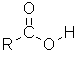Acid Anhydrides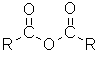Esters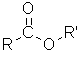Acyl Halides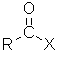AmidesNitriles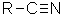Aldehydes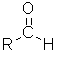Ketones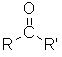Alcohols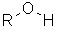Thiols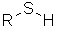Amines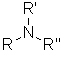Ethers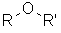Sulphides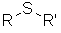Alkenes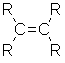Alkynes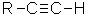Alkyl Halides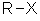Nitro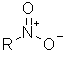Alkanes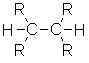© Dr. Ian Hunt, Department of Chemistry, University of Calgary